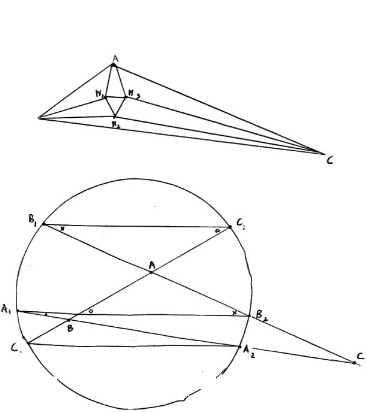(See the picture: angles o,x and . (this last symbol is a little dot!); Morley triangle M1M2M3) Since arc C2B2A2 = arc B1A1C1, we have o = x + . In triangle ABC the angle at A is pi - 2x - . , the angle at B is 2. + x, the angle at C is x - . With the horizontal lines, AB makes angle x + . , AC makes angle pi - x, BC makes angle pi - . , AM1 makes angle (x + .) + (pi - 2x - .)/3 = pi/3 + x/3 + 2./3 , etc. To prove that M1M3 is horizontal, we have to prove that the angle AM1M3 is equal to pi/3 + x/3 + 2./3 . Now to calculate this angle, knowing the angles of triangle ABC, is an exercise in analytic geometry.